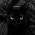## Wednesday, July 22, 2009

### Solution to the "Missing Square Problem"

Solution of the Missing Square Problem
Jason D.

Have you ever seen this "puzzle" floating around before?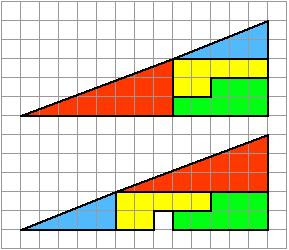This problem appears to make no sense what so ever…two triangles of apparently equal dimensions, consisting of smaller segments, appear to cover less area when rearranged differently...where did the missing block go?

Go ahead and try it for yourself (I used cut outs from graph paper as a starting point). Then come back and see if your solution agrees with mine!

The solution:

First, lets examine the larger rectangles. Each covers and area of (13 * 5)/2, or 32.5 square units.

The areas of the individual segements:

Blue: (2*5)/2 = 5 square units
Green: 8 square units
Yellow: 7 square units
Red: (3*8)/2 = 12 square units.

The sum? 32 square units. *GASP* neither triangle corresponds to the sum area of the segments.

In the top triangle, the area subtract the area of the yellow and green segments gives us 17.5 square units.

In the bottom triangle, this identical calculation yields 16.5 square units.

The different of course being *drum roll* one square unit. But WAIT! There's more.

The ratio of the full triangle is 13:5, the blue 5:2 and the red 8:3. These are NOT equivalent ratios.

We can look to our good friend Pythagoras:

Given the hypotenuse of each triangle is the square root of the sum of the other two sides squared, the hypotenuse of each triangle is:

Full Triangle: √194

Red: √73
Blue: √29

If this “triangle” is all that it seems, the hypotenuse of the red and blue triangle would equal that of the full triangle.

√73 = 8.5440037453175311678716483262397
+ √29 = 5.3851648071345040312507104915403
= 13.92916855245203519912235881778

√194 = 13.928388277184119338467738928513

So close! But the value is actually off by .00078027526791586065461988926674228

Lets look closely at the slopes.
Full Triangle : 5/13 = 0.38461538461538461538461538461538
Red Triangle : 3/8 = 0.375
Blue Triangle: 2/5 = 0.4

If this were what it looked like, then all the slopes would be equal. However, The blue triangle is steeper then the red, which is not as steep as the full triangle. This means that the hypotenuse formed by the two smaller triangles is not straight, and thus “slopes in” on one triangle and “slopes out” on the other.

The hypotenuse’s have different slopes, as the angles are different.

For n >= 5, this discrepancy is basically unnoticeable. But for n=4, n=3, you can see it quite clearly.From a different perspective:Still not convinced?Pretty nifty. But wait just a darn tootin' minute…look at those numbers… 1, 1, 2, 3, 5, 8, 13

Look familiar?

So if you’re still wondering where the missing block went, Fibonacci ate it.

The End.

1.Thanks, you did a fan-freakin tastic job of explaining it.

1.Thanks you for your explanation. My daugther and me enjoy it. ¡Great!
Thanks you very much.
(I am from Argentina)

2.very interesting! i had been searching for the answer to this problem for a long time now thanks!

3.fibonacci ate it xD ... nice conclussion to the problem... nice explanation

4.Thanks you for your explanation. My daugther and me enjoy it. ¡Great!
Thanks you very much.
(I am from Argentina)

5.Copperfield!

6.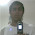Great explanation :)

Greetings from Argentina!

7.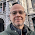Ohhhh, great explanation!, congratulations!

8.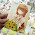Argentina = Taringa.
Gracias amigo

9.Who is "n" in your demostration?

1.and where did you get that sucesion of number for the Fibonacci series?

2.Hi,

Sorry for the late reply! N in this case is simply the number of vertical units (height on the Y-Axis).

The Fibonacci series comes from enumerating the dimensions of the inner segments. Hope that helps!

10.OMG what an explanation. But as a layman the answer is simple. The slopes of red and blue triangles are same in picture A. Similarly same in picture B too. Only the height of all the squares in picture B is lesser than that of picture A.

Therefore the gradients of picture A and B are not exactly the same!

Leaving the numbers aside it is obvious this illusion was achieved merely by changing the height of the squares in picture B.

11.